# Excel formula to count cells with certain text: exact and partial match

The tutorial shows how to count number of cells with certain text in Excel. You will find formula examples for exact match, partial match and filtered cells.

Last week we looked at how to count cells with text in Excel, meaning all cells with any text. When analyzing large chunks of information, you may also want to know how many cells contain specific text. This tutorial explains how to do it in a simple way.

## How to count cells with specific text in Excel

Microsoft Excel has a special function to conditionally count cells, the COUNTIF function. All you have to do is to supply the target text string in the criteria argument.

Here's a generic Excel formula to count number of cells containing specific text:

COUNTIF(range, "text")

The following example shows it in action. Supposing, you have a list of item IDs in A2:A10 and you want to count the number of cells with a particular id, say "AA-01". Type this string in the second argument, and you will get this simple formula:

`=COUNTIF(A2:A10, "AA-01")`

To enable your users to count cells with any given text without the need to modify the formula, input the text in a predefined cell, say D1, and supply the cell reference:

`=COUNTIF(A2:A10, D1)`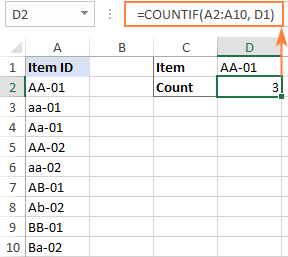Note. The Excel COUNTIF function is case-insensitive, meaning it does not differentiate letter case. To treat uppercase and lowercase characters differently, use this case-sensitive formula.

## How to count cells with certain text (partial match)

The formula discussed in the previous example matches the criteria exactly. If there is at least one different character in a cell, for instance an extra space in the end, that won't be an exact match and such a cell won't be counted.

To find the number of cells that contain certain text as part of their contents, use wildcard characters in your criteria, namely an asterisk (*) that represents any sequence or characters. Depending on your goal, a formula can look like one of the following.

Count cells that contain specific text at the very start:

COUNTIF(range, "text*")

Count cells that contain certain text in any position:

COUNTIF(range, "*text*")

For example, to find how many cells in the range A2:A10 begin with "AA", use this formula:

`=COUNTIF(A2:A10, "AA*")`

To get the count of cells containing "AA" in any position, use this one:

`=COUNTIF(A2:A10, "*AA*")`

To make the formulas more dynamic, replace the hardcoded strings with cell references.

To count cells that begin with certain text:

`=COUNTIF(A2:A10, D1&"*")`

To count cells with certain text anywhere in them:

`=COUNTIF(A2:A10, "*"&D1&"*")`

The screenshot below shows the results: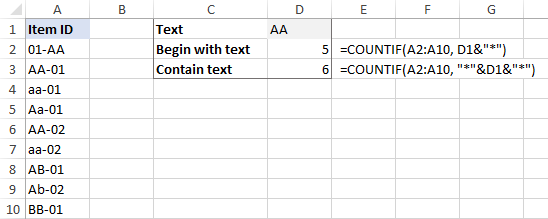## Count cells that contain specific text (case-sensitive)

In situation when you need to differentiate uppercase and lowercase characters, the COUNTIF function won't work. Depending on whether you are looking for an exact or partial match, you will have to build a different formula.

### Case-sensitive formula to count cells with specific text (exact match)

To count the number of cells with certain text recognizing the text case, we will use a combination of the SUMPRODUCT and EXACT functions:

SUMPRODUCT(--EXACT("text", range))

How this formula works:

• EXACT compares each cell in the range against the sample text and returns an array of TRUE and FALSE values, TRUE representing exact matches and FALSE all other cells. A double hyphen (called a double unary) coerces TRUE and FALSE into 1's and 0's.
• SUMPRODUCT sums all the elements of the array. That sum is the number of 1's, which is the number of matches.

For example, to get the number of cells in A2:A10 that contain the text in D1 and handle uppercase and lowercase as different characters, use this formula:

`=SUMPRODUCT(--EXACT(D1, A2:A10))`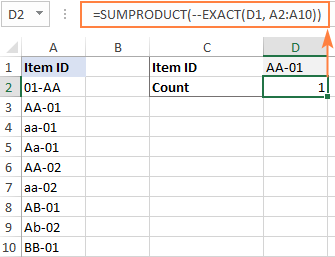### Case-sensitive formula to count cells with specific text (partial match)

To build a case-sensitive formula that can find a text string of interest anywhere in a cell, we are using 3 different functions:

SUMPRODUCT(--(ISNUMBER(FIND("text", range))))

How this formula works:

• The case-sensitive FIND function searches for the target text in each cell of the range. If it succeeds, the function returns the position of the first character, otherwise the #VALUE! error. For the sake of clarity, we do not need to know the exact position, any number (as opposed to error) means that the cell contains the target text.
• The ISNUMBER function handles the array of numbers and errors returned by FIND and converts the numbers to TRUE and anything else to FALSE. A double unary (--) coerces the logical values into ones and zeros.
• SUMPRODUCT sums the array of 1's and 0's and returns the count of cells that contain the specified text as part of their contents.

To test the formula on real-life data, let's find how many cells in A2:A10 contain the substring input in D1:

`=SUMPRODUCT(--(ISNUMBER(FIND(D1, A2:A10))))`

And this returns a count of 3 (cells A2, A3 and A6):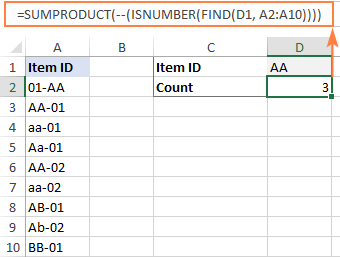## How to count filtered cells with specific text

To count visible items in a filtered list, you will need to use a combination of 4 or more functions depending on whether you want an exact or partial match. To make the examples easier to follow, let's take a quick look at the source data first.

Assuming, you have a table with Order IDs in column B and Quantity in column C like shown in the image below. For the moment, you are interested only in quantities greater than 1 and you filtered your table accordingly. The question is – how do you count filtered cells with a particular id?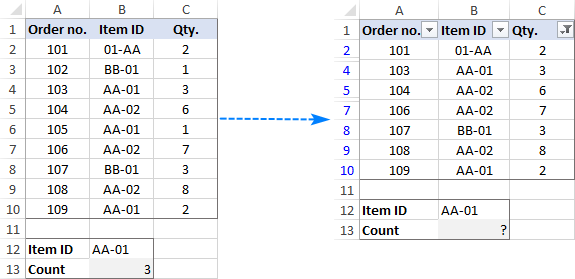### Formula to count filtered cells with specific text (exact match)

To count filtered cells whose contents match the sample text string exactly, use one of the following formulas:

`=SUMPRODUCT(SUBTOTAL(103, INDIRECT("A"&ROW(A2:A10))), --(B2:B10=F1))`

`=SUMPRODUCT(SUBTOTAL(103, OFFSET(A2:A10, ROW(A2:A10) - MIN(ROW(A2:A10)),,1)), --(B2:B10=F1))`

Where F1 is the sample text and B2:B10 are the cells to count.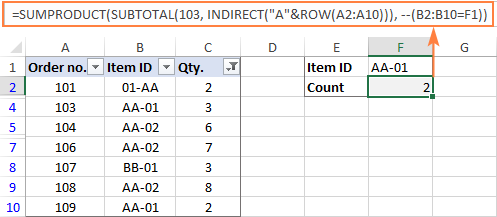How these formulas work:

At the core of both formulas, you perform 2 checks:

1. Identify visible and hidden rows. For this, you use the SUBTOTAL function with the function_num argument set to 103. To supply all the individual cell references to SUBTOTAL, utilize either INDIRECT (in the first formula) or a combination of OFFSET, ROW and MIN (in the second formula). Since we aim to locate visible and hidden rows, it does not really matter which column to reference (A in our example). The result of this operation is an array of 1's and 0's where ones represent visible rows and zeros - hidden rows.
2. Find cells containing given text. For this, compare the sample text (F1) against the range of cells (B2:B10). The result of this operation is an array of TRUE and FALSE values, which are coerced to 1's and 0's with the help of the double unary operator.

Finally, the SUMPRODUCT function multiplies the elements of the two arrays in the same positions, and then sums the resulting array. Because multiplying by zero gives zero, only the cells that have 1 in both arrays have 1 in the final array. The sum of 1's is the number of filtered cells that contain the specified text.

### Formula to count filtered cells with specific text (partial match)

To count filtered cells containing certain text as part of the cell contents, modify the above formulas in the following way. Instead of comparing the sample text against the range of cells, search for the target text by using ISNUMBER and FIND as explained in one of the previous examples:

`=SUMPRODUCT(SUBTOTAL(103, INDIRECT("A"&ROW(A2:A10))), --(ISNUMBER(FIND(F1, B2:B10))))`

`=SUMPRODUCT(SUBTOTAL(103, OFFSET(A2:A10, ROW(A2:A10) - MIN(ROW(A2:A10)),,1)), --(ISNUMBER(FIND(F1, B2:B10))))`

As the result, the formulas will locate a given text string in any position in a cell: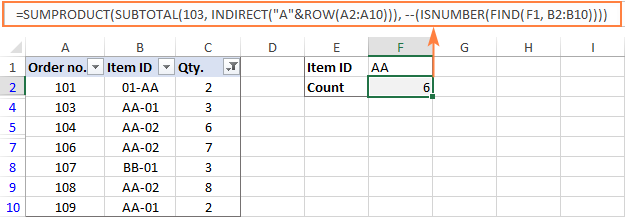Note. The SUBTOTAL function with 103 in the function_num argument, identifies all hidden cells, filtered out and hidden manually. As the result, the above formulas count only visible cells regardless of how invisible cells were hidden. To exclude only filtered out cells but include the ones hidden manually, use 3 for function_num.

That's how to count the number of cells with certain text in Excel. I thank you for reading and hope to see you on our blog next week!

Excel formulas to count cells with certain text

### 20 responses to "Excel formula to count cells with certain text: exact and partial match"

1.Laiba says:

Fail+Pass+Pass = PASS
Pass+Fail+Pass = PASS
Pass+Pass+Fail = FAIL
Fail+Pass+Pass = FAIL
HOW ??????
Help me to solve this.

2.Matthew Turney says:

Is there a countif formula to count cells if they do NOT contain specific words. For example, I have a countif formula for everything that contains "BAW" in it. Now I would like a countif formula for everything except "BAW" in it but I need to be able to address multiple words like {"BAW","BAE"} etc. Any help would be appreciated. Thank you.

•Alexander Trifuntov (Ablebits.com Team) says:

Hello Matthew!
If I understand your task correctly, the following formula should work for you:
=SUMPRODUCT(--(\$A\$1:\$A\$17<>\$E\$1),--(\$A\$1:\$A\$17<>\$E\$2))
where in E1 written "BAE", in E2 - "BAW"

3.Lori-Ann says:

Thank you for your tutorial it was very insightful and helpful to me. I am wondering though if there is a countif formula that will count letters and numbers and then total the numbers? For Example: if I have cells that contain V10, V8, F8, F4, and V2 is there a countif formula that would total the numbers with the V (10+8+2=20)and the F (8+4) and then total the cells so they read V20 or F10?
I do not know if that is even possible but I am hoping that it is.
Thank you very much for your time

•Alexander Trifuntov (Ablebits.com Team) says:

Hello Lori-Ann!
If I understand your task correctly, the following formula should work for you:

=CONCATENATE("V",SUMPRODUCT(--(LEFT(A1:A16,1)="V"), --(IFERROR(RIGHT(A1:A16,LEN(A1:A16)-1),0))))

I hope this will help, otherwise please do not hesitate to contact me anytime.

4.Bianca Schurink says:

Hi
I'm looking for a formula that if column F says 'VA' then the numeric number in the same row but column E needs to be added into a total on a table.
If the cell in column F is changed to a different variable eg 'OS' then the value is subtracted from the 'VA' total and added to the 'OS' total.

•Alexander Trifuntov (Ablebits.com Team) says:

Hello Bianca!
I’m sorry but your task is not entirely clear to me. For me to be able to help you better, please describe your task in more detail. Please specify what you were trying to find, what formula you used and what problem or error occurred. Give an example of the source data and the expected result.
It’ll help me understand it better and find a solution for you. Thank you.

5.Richard McWhorter says:

Hello,

I like your tutorials! Very clear and direct. I am trying to sort thru a column containing ship to information. Specifically I am wanting to count the number of orders that were shipped to each state. Sometimes the Stated is spelled out, say Georgia. Other times it is abbreviated, say GA. I can use countif with a wildcard, but then I need create a formula for each state. Is there an easy way to review the range, group it by state and then count the results? Thanks in advance for any help you can provide!

6.Chantal says:

Hi. I have a worksheet with numerous text and numbers. In column A I have different text:A1 AAAA A2: BBBB: A3: AAAA as well. In column be I have numbers B1:1,B2:2,B3:1. How do I lookup for AAAA in column and then count the values which is picked up in column B?

•Alexander Trifuntov (Ablebits.com Team) says:

Hello!
The formula below will do the trick for you:

=SUMPRODUCT(--("AAA"=A1:A15),B1:B15)

•Chantal says:

Thanks so much.
I have another problem....
I have also a sheet with multiple texts and 2 sheets.
On sheet 1 I have text AAA in column A
On sheet 2 I have various lines in
Column A Column B Column C
AAA CAT 1
BBB DOG 1
BBB CAT 1
AAA CAT 2
How do I look up only AAA Cat on sheet 2 and count the CAT in various lines so that CAT shows the amount of 3 in sheet 1 for AAA.
Apologies I'm only entry into Excel :-)

•Alexander Trifuntov (Ablebits.com Team) says:

Hello!
To count the number of lines containing both "AAA" and "CAT", use the formula

=SUM(--("AAACAT"=A1:A15&B1:B15))

7.Marin says:

Good afternoon,
I have last names in cells in column c. Task is to count all cells in range that contains letter "v" on second place. Would You be so kind and give me solution.

•Alexander Trifuntov (Ablebits.com Team) says:

Hello!
If I understand your task correctly, the following formula should work for you:

=SUM(--(MID(A1:A10,2,1)="v"))

I hope this will help

8.PoppyF says:

Hi - I'm looking for a formula which will help me with the following:

To return a value (P for example) in a cell when there is a name within the text of a cell (the text will involve other words than just the name, as it's a description) in a different column that corresponds to a name within a list in a different sheet of the workbook. Multiple names within the list which all need to be checked against what comes up in the cells I'm searching to return a value from.

Any help will be hugely appreciated! Many thanks

•Alexander Trifuntov (Ablebits.com Team) says:

Hello!
9.Jacques Boyde Panteria Paragas says:
10.Vibha says: